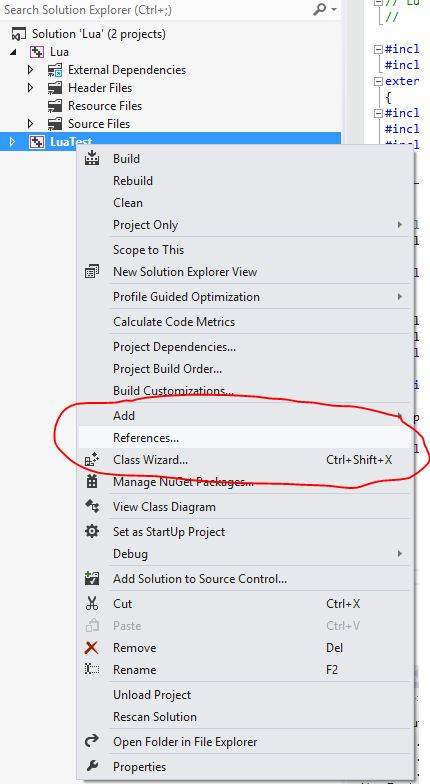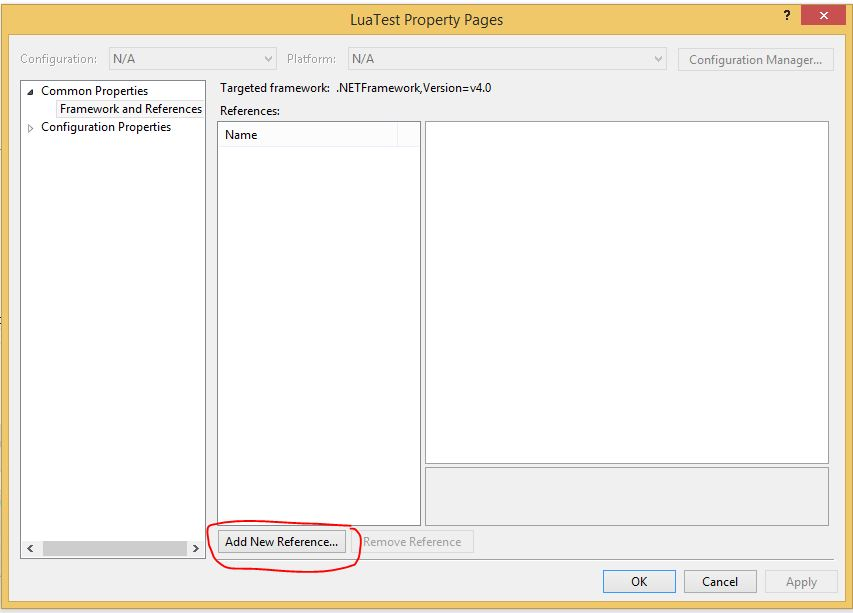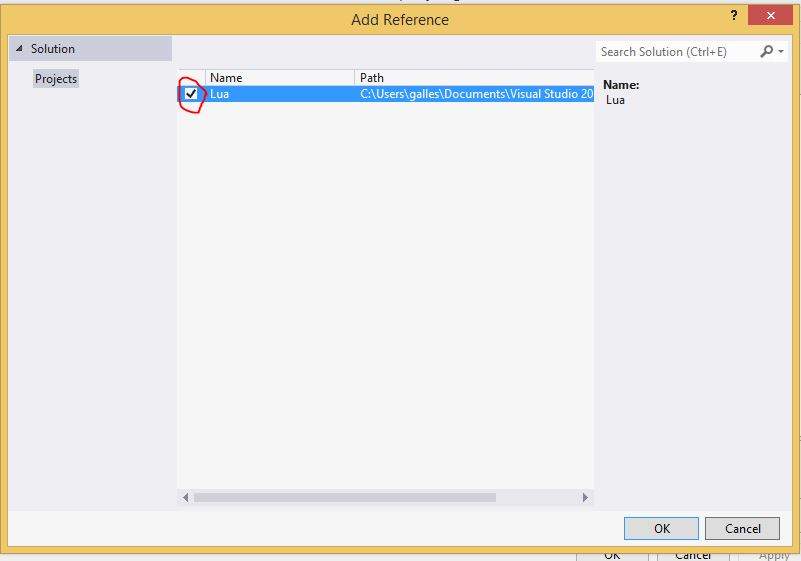# Compiling Lua

The lua source can be found at the lua website here. We'll be using the latest version, 5.2.3 (though other recent versions of Lua will behave similarly).

Once you have the latest lua source, it's time to build it.  (Yes, you could just download header files and the .dll, but what's the fun in that?  When available, it's always better to have the source, that way you can see how things are really working -- and you can debug more easily).   Using visual studio:

• Open up Visual Studio, and create a new project
• Create a win 32 console application
• In the Project Creation wizard, check the "static library" radio button to build as a static library.
• Add the lua source to the project. Just dragging the files directly from their folders into the project works well for this.
• There are two source files that you don't need: lua.c and luac.c. These files contain the main program for the command line interpreter and the bytecode compiler, respectively. You can remove this files from the project if you wish. If you are building as a static library, having more than one main won't be a problem. However, if you are building as an executable, then you will need to exclude one of these files from the project. Right-click on them in the project explorer and select "Exclude from Project"

You now have a project that you can add to any solution that needs lua. Once you have added the lua project to your game, you need to do a few more steps:

• In the properties for the project that is using lua, be sure that the directory that contains the lua .h files is included in the set of include paths. If at all possible, make these paths relative, and not absolute
• Your project will need to get at the .lib files for the lua. There are a few ways to do this:
• Add a reference from your project to the lua project. Right-click on your project in the project explorer, and select References:Select the lua project:• Or, add Lua.lib to "Additional Dependencies" (in the Librarian/Genrerl tab of the Project Property Pages) and add the directory where Lua.lib lives to "Additional Library Directories" (also in the Librarian/Genrerl tab of the Project Property Pages).

Once we have build Lua, and set up our project dependencies correctly, we are ready to use in on our application,

# Setting up and shuting down Lua interpreter

First off, we need to include the proper header files.  Since Lua is ANSI C, if we are coding in C++, we will need to enclose the #includes in extern "C":

```extern "C"
{
#include "lua.h"
#include "lualib.h"
#include "lauxlib.h"
}
```

Everything that we need to mantain the current state of the interprer (all global tables, etc) is stored in a variable of type lua_State.  The first thing we need to do is create an initial state (essentially, an instantiation of the lua interpreter), which is done by the call:

```lua_State* L = luaL_newstate();
```

Every time we want to access the interpreter, we need to pass in this state variable.  We can actually have as many instances of Lua state variables as we like (for instance, if we wanted to run separate instances of the interpreter on separate processors, or we wanted different scripts to have different global namespaces), though typically people just use one instance of the interpreter that all scripts use. The global tables in this state variable contain all "core" functions, but none of the libraries.  The libraries contain a bunch of important functions -- including everything in math, and i/o functions like print -- so we'd like to load them.  We can load libraries with the call:

```luaL_openlibs(L);
```
```Once we are done, we can free up all the space in the Lua state with
the call:```
```lua_close(L);
```

So, now we have the skeleton of a main function:

```extern "C"
{
#include "lua.h"
#include "lualib.h"
#include "lauxlib.h"

}

int main(int argc, char *argv[])
{

lua_State* L;

// initialize Lua interpreter
L = luaL_newstate();

// load Lua base libraries (print / math / etc)
luaL_openlibs(L);

////////////////////////////////////////////
// We can use Lua here !
/////////////////////////////////////////////

// Cleanup:  Deallocate all space assocatated with the lua state */
lua_close(L);

// Hack to prevent program from ending immediately
printf( "Press enter to exit..." );
getchar();

return 0;

}
```

We are ready to start using lua!

# Executing Lua files

We will start with the simplest way to use lua -- have the interpreter execute a file.  This is functionally equivalent to the dofile command from within lua (and unsurprisingly, has the same name!).  To execute the file "test.lua", we can use the call:

```    luaL_dofile(L, "myFile.lua");
```

Note that if we are using relative paths other than absolute paths under windows, then the system will look in the current working directory -- which is the directory that the executable file is in (if you double-click on the executable), or the directory that the project is in (if you run from within Visual Studio)  You can, of course, change the working directory that the debugger uses under the project settings in Visual Studio.

So, if we use the following myFile.lua:

```function fib(n)
if n == 1 or n == 2 then
return 1,1
end
prev, prevPrev = fib(n-1)
return prev+prevPrev, prev
end

print(fib(5))
print((fib(10)))
```

when the command luaL_dofile(L, "myFile.lua") is executed, the following will be printed out

```5           3
55
```

Note that the dofile command not only computes these values and prints them out, it also adds the fib function to the global namespace of our lua enviornment (stored in the C variable L).

# Calling Lua functions from C/C++

We can also call lua functions directly from C/C++, and get back the return values to use in our C/C++ code. To call a lua function we:

• Push the function on the top of the lua stack, using a call to
• lua_getGlobal(lua_state *L, char *globalName)
• Push the arguments to the function on the lua stack, using the functions:
• lua_pushnumber(lua_state *L, float number)
• lua_pushstring(lua_state *L, char *str)
• lua_pushboolean(lua_state *L, bool)
• lua_pushuserdata(lua_state *L, void *userdata)
• lua_pushnil(lua_state *L)
• Call the function, using the function:
• lua_call(lua_state *L, int numArguments, int numReturnValues)

We need to pass in both the number of arguments that we are passing in, and the number of arguments that we expect in return. Lua is very loose about the number of arguments and return value, but C/C++ is less so -- hence we need to be explicit when calling lua from C/C++.

• Extract the return values, using the functions:

• int lua_tointeger(lua_state *L, int stackLocation)

Grab an integer value off the lua stack. The first return value is at index -1, the second return value is at index -2, and so on.

• double lua_tonumber(lua_state *L, int stackLocation)

Grab a double value off the lua stack. The first return value is at index -1, the second return value is at index -2, and so on.

• const char *lua_tolstring(lua_state *L, int stackLocation)

Grab a string value of the lua stack

• int lua_toboolean(lua_state *L, int stackLocation)

Grab a boolean value off the lua stack

Note that these functions "peek" at the lua stack, they don't actually remove any values from the stack.  (That is done by the next function)

• Pop the return value(s) off the top of the sack (this is just to clean up) with a call to

• lua_pop(lua_state *L, int numReturnValsToPop);

Let's take a look at how we might call the fib function defined in the previous section to find the 13th Fibonacci number:
```
// Push the fib function on the top of the lua stack
lua_getglobal(L, "fib");

// Push the argument (the number 13) on the stack
lua_pushnumber(L, 13);

// call the function with 1 argument, returning a single result.  Note that the function actually
// returns 2 results -- we just want one of them.  The second result will *not* be pushed on the
// lua stack, so we don't need to clean up after it
lua_call(L, 1, 1);

// Get the result from the lua stack
int result = (int)lua_tointeger(L, -1);

// Clean up.  If we don't do this last step, we'll leak stack memory.
lua_pop(L, 1);
```

Let's look at a second example.  Assume that we had defined the following lua function add (that we could define by calling lua_dofile):

```add = function(a,b)
return a + b
end
```

We could call this function to add from C/C++ with the code:

```
int luaAdd(lua_State* L, int a, int b)
{

// Push the add function on the top of the lua stack

// Push the first argument on the top of the lua stack
lua_pushnumber(L, a);

// Push the second argument on the top of the lua stack
lua_pushnumber(L, b);

// Call the function with 2 arguments, returning 1 result
lua_call(L, 2, 1);

// Get the result
int sum = (int)lua_tointeger(L, -1);

// The one result that was returned needs to be popped off.  If the 3rd
//  parameter to lua_call was larger than 1, we would need to pop off more
//  elements from the lua stack.
lua_pop(L, 1);

return sum;
}
```

# Calling C/C++ functions from Lua

So now you could write scripts that you can call from your game engine.  However, these scripts won't be very useful if they can't interact with your game objects -- you need a way for your scripts to affect the game world.  To do that, you need to be able to call C/C++ functions from lua.  Since Lua and C++ have very different conventions for how functions are called, there is a little bit of wrapper work that needs to be done.  First, write a C function that takes as input a Lua state variable (the parameters for the function will need to be extracted by hand from the lua stack -- this is slightly tedious, but not too difficult.)  Then, the function will need to be registered with lua.

## Step 1:  Writing a C function that Lua can call

C/C++ functions that are called from lua need to take as an input parameter the lua state.  The parameters can be examined on the call stack using the functions lua_tointeger, lua_tonumber, etc (described above).  The first parameter is at index 1, the second parameter is at index 2, and so on.  Once we have extracted the parameters, we do our calculation, and then push the result on the top of the stack.

```int cAdd(lua_State *L)
{
// Step 1:  extract the parameters from the lua stack:
double n1 = lua_tonumber(L,1);
double n2 = lua_tonumber(L,2);

//  Step 2:  Do the actual calculation.  Normally, this will be more interesting than a single sum!
double sum = n1 + n2;

// Step 3:  Push the result on the lua stack.
lua_pushnumber(L,sum);

// Return the number of arguments we pushed onto the stack (that is, the number of return values this
// function has
return 1;
}
```
Let's look at a slightly more complicated C function.  We can write C functions that takes a variable number of parameters, and returns more than one return value.  While the previous function assumed that we were passed in two parameters, we can instead query the lua state to see how many parameters were actually passed into the function.  The number of parameters is stored on the top of the stack, which can be accessed by a call to lua_gettop(lua_state *L).  Let's look at a function that takes in multiple parameters, and calculates the sum and average of all parameters that were passed in:

```int average(lua_State *L)
{
// Get the number of parameters
int n = lua_gettop(L);

double sum = 0;
int i;

// loop through each argument, adding them up
for (i = 1; i <= n; i++)
{
sum += lua_tonumber(L, i);
}

// push the average on the lua stack
lua_pushnumber(L, sum / n);

// push the sum on the lua stack
lua_pushnumber(L, sum);

// return the number of results we pushed on the stack
return 2;
}
```

## Step 2:  Registering the C function for lua

Once we have written the function, we just need to register it with lua.  That is, we need to add the name of the function to the global lua namespace, and provide a pointer to the function so that lua cal access it.   There is a helpful macro for this:     lua_register(lua_state *L, const char *name, functionPointer fn).  So, to register the above two functions:

```    lua_register(L, "cAdd", cAdd);
lua_register(L, "average", average);
```

## Step 3:  Calling the C funcion from lua

This part is easy -- once the function is registered, lua can call it like any other function.

So, the complete round trip is:

• Start the lua interpreter
• Register the C functions you want lua to use
• Call a lua function from within C, either by a call to  luaL_dofile, or by calling a lua function directly
• The lua function that you called from C can access the C function

# Executing Lua Strings

We can also send a string straight to the lua interpreter, and it will be executed just as if that string was in a file that was executed with a dofile.  So, we could do something like:

```luaL_dostring(L, "for x = 1, 5 do print(x) end");
```

and we would get the output:

```1
2
3
4
5
```

We could thus create a braindead interpreter as follows:

```    while (true)
{
printf(">");
fgets(inputBuffer, sizeof inputBuffer, stdin);
luaL_dostring(L, inputBuffer);
}
```

Note that this would not work at all in a game environment! We will look at how to embed a command-line lua interpreter within a game next time. For now, this is enough for us to play around with a bit.

# Practical Exercise

Now we are ready to get our fingers dirty!

1. Download the following project, which contains a basic skeleton lua framework
2. Write a function in C (exposed to Lua, much like average and cAdd) that takes as input an integer n, and returns all prime numbers less than or equal to n.
• Test your primes function in the interpreter with "print(primes(100))"
3. Write a function in lua nthPrime, that takes as input a number n, and returns the nth prime number. So, nthPrime(6) should return 13. We'll define nthPrime(1) to be 2, and nthPrime(0) will be undefined. Your lua function should call the c primes function
• Small change in how lua does variable numbers of arguments: From the notes
```sum = function(...)
local result = 0
for i,v in ipairs(arg) do
result = result + v
end
return result
end
```

Alas, this does not work. But we can fix it with a simple change:

```sum = function(...)
local result = 0
local arg = { ... }
for i,v in ipairs(arg) do
result = result + v
end
return result
end
```
4. Write C code that computes the 100th prime number by calling the lua nthPrime function, and prints the value out using printf. So, C calling Lua that calls C.